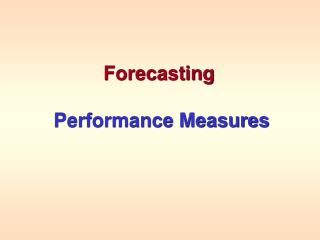DownloadDownload PresentationForecasting Performance Measures

# Forecasting Performance Measures

Télécharger la présentation## Forecasting Performance Measures

- - - - - - - - - - - - - - - - - - - - - - - - - - - E N D - - - - - - - - - - - - - - - - - - - - - - - - - - -
##### Presentation Transcript

1. Forecasting Performance Measures

2. Comparing Forecasting Techniques • There is more than one approach that can be used to generate a forecast from a set of time series values. • For the stationary Yoho model we used: • Last Period • 4-Period Moving Average • 4-Period Weighted (.4, .3, .2, .1) Moving Average • Question: “Which method appears to be the most effective for a particular set of time series values” Answer – The one that comes “the closest” on the average if used to forecast values of the current time series.

3. Forecast Errors What was forecasted to happen at time t using the forecast technique Forecast Error At time t What actually happened at time t To try to determine which one of these forecasting methods gives the “best” forecast, we evaluate the “success” of each method using a performance measure. Each performance measure begins by evaluating the forecast errors tfor each time period t given by: t = yt - Ft

4. Performance Measures S |Dt| n yt n S(D t)2 n MAPE = MSE = |D t| n S MAD = |D t| LAD = max Mean Absolute Percent Error MAPE Mean Square Error MSE Mean Absolute Deviation MAD Largest Absolute Deviation LAD

5. Which Performance Measure Should Be Used? • Choice of the modeler • Advantages • MSE gives greater weight to larger deviations (which could result from outliers) • MAD gives less weight to larger deviations • MAPE gives less overall weight to a large deviation if the time series value is large • LAD tells us if all deviations fall below some threshold value • Although we illustrate all 4 techniques, in general focus will be on MSE and MAD.

6. Excel – Performance Measures for Last Period Technique =ABS(D3)/B3 =ABS(D3) =B3-C3 =D3^2 Drag cells D3:G3 down to D53:G53 =AVERAGE(E3:E53) =AVERAGE(F3:F53) =AVERAGE(G3:G53) Note: Rows 8-43 are hidden =MAX(F3:F53)

7. Excel – Performance Measures for 4-Period Moving Average Technique =ABS(D6)/B6 =ABS(D6) =B6-C6 =D6^2 Drag cells D6:G6 down to D53:G53 =AVERAGE(E6:E53) =AVERAGE(F6:F53) =AVERAGE(G6:G53) Note: Rows 8-43 are hidden =MAX(F6:F53)

8. Performance Measures for 4-Period Weighted Moving Average Technique =ABS(D6)/B6 =ABS(D6) =B6-C6 =D6^2 Drag cells D6:G6 down to D53:G53 =AVERAGE(E6:E53) =AVERAGE(F6:F53) =AVERAGE(G6:G53) Note: Rows 8-43 are hidden =MAX(F6:F53)

9. Performance MeasuresSummary MSE MAD MAPE LAD Last Period 19631 110.96 32.90 359.00 4-Period MA 11037 88.75 25.61 223.25 4-Period WMA 11992 92.39 26.78 258.40 4-Period Moving Average performed best using all performance measures. This is not all ways the case.

10. Review • Performance Measures allow the comparison of the accuracy of various forecasting techniques applied to a particular time series. • Performance Measures are all based on deviations of forecasted values from actual values in the time series: Δt = yt- Ft • MSE averages the squared deviations • MAD averages the absolute deviations • MAPE averages the absolute percent errors • LAD is the largest absolute deviation • Excel